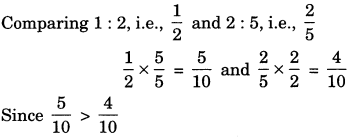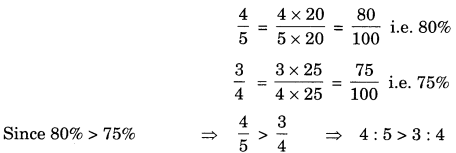On this page, you will find Comparing Quantities Class 7 Notes Maths Chapter 8 Pdf free download. CBSE NCERT Class 7 Maths Notes Chapter 8 Comparing Quantities will seemingly help them to revise the important concepts in less time.

## CBSE Class 7 Maths Chapter 8 Notes Comparing Quantities

### Comparing Quantities Class 7 Notes Conceptual Facts

1. Ratio: Comparison of two quantities of same kind and with same unit is called ratio.
For example: a : b or $$\frac{a}{b}$$ where a is called Antecedent and b Consequent. b

2. Ratio in simplest form: A ratio is said to be in simplest form if its antecedent and consequent have no common factor other than.
For example: $$\frac{2}{3}, \frac{3}{7}, \frac{2}{5}, \frac{6}{7}$$ etc. or 2 : 3, 3 : 7, 2 : 5 and 6 : 7 etc.

3. Equivalent ratios: Two ratios can be compared by converting then into like fractions. If the two factions are equal, then they are called as equivalent ratio.
For example: 15 : 20 is equivalent to 3 : 4.
Check whether 1: 2 and 2 : 5 are equivalent.∴ 1 : 2 and 2 : 5 are not equivalent ratios.

4. Comparison of ratios: Let us take from two ratios 2 : 3 and 4 : 5
2 : 3 = $$\frac{2}{3}$$ and 4 : 5 $$\frac{2}{3}$$
= 2 x 5 and 3 x 4
2 x 5 and 3 x 4 (By Cross-multiplicative)
10 and 12
$$10<12 \Rightarrow \frac{2}{3}<\frac{4}{5}$$
Hence 2:3< 4:5
We can also compare more than two ratios.

5. Percentage: Ratios can also be compared by converting it into percent i.e. per hundred.
For example:
Let us take two ratios $$\frac{4}{5} \text { and } \frac{3}{4}$$ converting into Percentage, we have6. Proportion: When two ratios are equivalent, then the four quantities are in proportion.
Let a : b and c : d are equivalent ratios
a : b :: c : d      [:: Symbol of proportion]
$$\frac{a}{b}=\frac{c}{d}$$ ⇒ a x d = c x b
a and d are called extremes and b and c are called means
∴ Product of extremes = Product of means

7. Continued proportion: If a, b and c be three quantities such that a: b:: b: c, then a, b, c are in continued proportion.
$$\frac{a}{b}=\frac{b}{c} \quad \Rightarrow \quad b^{2}=a c \Rightarrow b=\sqrt{a c}$$

8. Unitary method: In this method, we find the value of unit quantity and then the value of required quantity is calculated. There are two types of variation.

• Direction variation
• Inverse variation

9. Conversion of a fraction into percent: To convert $$\frac{2}{5}$$ into percent, we have
$$\frac{2}{5}$$ x 100% =40%

10. Conversion of percent into fraction: To convert 20% into fraction, we have
20% = $$\frac{20}{100}=\frac{1}{5}$$

11. Conversion of a ratio into per cent: To convert 4 : 5 into per cent, we have
4:5= $$\frac{4}{5}$$ x 100% = 80%

12. Conversion of a percent into ratio: To convert 75% into ratio, we have
75% = $$\frac{75}{100}=\frac{3}{4}$$ i.e, 3:4

13. Simple interest:[Here SP means selling price and CP means cost price]Profit and Loss per cent are always calculated on CP.